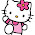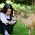## Friday, December 11, 2015

### SECCON 2015 - Nonogram - 300 point Programming Challenge

Short break but we're back CTFing. I've been away because I took a month of lab time doing the OSCP certification which I will write a review of later. It was very fun though. Today I'm writing up how I solved this challenge at SecCon 2015 since it was one I spent some non-trivial time on.

A nonogram is a type of Japanese crossword type puzzle with numbers. You fill in boxes based on numbers in the rows and columns and eventually make a picture.

The SecCon implementation looked like this (NO! added for comedic effect :P ):

I looked into solving the puzzle category itself but grew tired of reading the rules and instead looked at solving engines other people had written.

I first selected this one from Github. The solver worked and was simple to integrate with some simple network code but it was very slow and often tried to perform useless exhaustive searches for perfect solutions which just didn't ever seem to end.

That was where I spent most of my time on this challenge, diagnosing why Nonograms take time to solve. I found that there's often more than one solution and sometimes there are many possible solutions.

So I went back on the hunt for a better Nonogram solver and came across this article about using Google CP solver for faster Nonogram solutions using DefaultSearch.

Sounded good and, again was in Python, so I basically swapped out the old engine of my solution for this one in a couple of minutes and immediately saw results. The solver was way faster and knew how to give up after finding 2 (or, modified as many as you like) possible solutions.

With that engine, some very rough web and table parsing code, and we had a solver for this challenge that would:

1. Grab the first / next challenge nonogram table
2. Parse for the values in the first column and top row into two zero padded lists
3. Feed that into the Google constraint programming engine via some borrowed Python code
4. Get a text output, write that using Python Image Library to a PNG file
5. Parse the PNG for a QR code
6. Send the QR code decoded output to the server and fetch the next challenge
We had to do this a total of 30 times and the solutions aren't always perfect and the QR codes don't always work so a few attempts need to be run.

Anyway, I include the code before, sorry you have to see this messy hack but I don't mind, 300 points it got me :)

```#!/usr/bin/python
# -*- coding: utf-8 -*-

import requests
import re
from itertools import izip
import PIL
from PIL import ImageFont,Image,ImageDraw
import subprocess
import sys
from ortools.constraint_solver import pywrapcp

URL = 'http://qrlogic.pwn.seccon.jp:10080/game/'

#
# Make a transition (automaton) list of tuples from a
# single pattern, e.g. [3,2,1]
#
def make_transition_tuples(pattern):
p_len = len(pattern)
num_states = p_len + sum(pattern)

tuples = []

# this is for handling 0-clues. It generates
# just the minimal state
if num_states == 0:
tuples.append((1, 0, 1))
return (tuples, 1)

# convert pattern to a 0/1 pattern for easy handling of
# the states
tmp = 
c = 0
for pattern_index in range(p_len):
tmp.extend( * pattern[pattern_index])
tmp.append(0)

for i in range(num_states):
state = i + 1
if tmp[i] == 0:
tuples.append((state, 0, state))
tuples.append((state, 1, state + 1))
else:
if i < num_states - 1:
if tmp[i + 1] == 1:
tuples.append((state, 1, state + 1))
else:
tuples.append((state, 0, state + 1))
tuples.append((num_states, 0, num_states))
return (tuples, num_states)

#
# check each rule by creating an automaton and transition constraint.
#
def check_rule(rules, y):
cleaned_rule = [rules[i] for i in range(len(rules)) if rules[i] > 0]
(transition_tuples, last_state) = make_transition_tuples(cleaned_rule)

initial_state = 1
accepting_states = [last_state]

solver = y.solver()
transition_tuples,
initial_state,
accepting_states))

def silly(rows, row_rule_len, row_rules, cols, col_rule_len, col_rules):
font = ImageFont.truetype('clacon.ttf')
img = Image.new("RGBA", (300,1500),(255,255,255))
draw = ImageDraw.Draw(img)
y_text = 8

# Create the solver.
solver = pywrapcp.Solver('Nonogram')

#
# variables
#
board = {}
for i in range(rows):
for j in range(cols):
board[i, j] = solver.IntVar(0, 1, 'board[%i, %i]' % (i, j))

board_flat = [board[i, j] for i in range(rows) for j in range(cols)]

# Flattened board for labeling.
# This labeling was inspired by a suggestion from
# Pascal Van Hentenryck about my (hakank's) Comet
# nonogram model.
board_label = []
if rows * row_rule_len < cols * col_rule_len:
for i in range(rows):
for j in range(cols):
board_label.append(board[i, j])
else:
for j in range(cols):
for i in range(rows):
board_label.append(board[i, j])

#
# constraints
#
for i in range(rows):
check_rule(row_rules[i], [board[i, j] for j in range(cols)])

for j in range(cols):
check_rule(col_rules[j], [board[i, j] for i in range(rows)])

#
# solution and search
#
parameters = pywrapcp.DefaultPhaseParameters()
parameters.heuristic_period = 200000

db = solver.DefaultPhase(board_label, parameters)

solver.NewSearch(db)

num_solutions = 0
while solver.NextSolution():
num_solutions += 1
for i in range(rows):
row = [board[i, j].Value() for j in range(cols)]
row_pres = []
for j in row:
if j == 1:
row_pres.append('█')
else:
row_pres.append(' ')
outline = ''.join(row_pres)
outline = unicode(outline,"utf-8")
width, height = font.getsize(outline)
draw.text((0,y_text),outline,(0,0,0),font=font)
y_text += height
draw = ImageDraw.Draw(img)

draw.text((0,y_text)," ",(0,0,0),font=font)
y_text += height
draw.text((0,y_text)," ",(0,0,0),font=font)
y_text += height
draw.text((0,y_text)," ",(0,0,0),font=font)
y_text += height

if num_solutions >= 6:
break

solver.EndSearch()
img = img.resize((700,2000))
img.show()

print "[*] Posting result: " + answer
payload = { 'ans' : answer }
r = s.post(URL, data=payload)
else:
print "[*] Using GET Method to get first challenge..."
r = s.get(URL)

toprow = []
firstcolumn = []

for line in r.content.splitlines():
if 'Stage:' in line:
thestage = line.split(" ")
print "[*] Stage: " + thestage + " / 30"

if '<th class="cols">' in line:
p = re.compile("<span>(.*?)</span>")
for block in headerblocks:
columnnums = []
if '<th class="rows">' in block:
break

subblocks = block.split("<br />")
for subblock in subblocks:
m = p.search(subblock)
if m:
columnnums.append(int(m.group(1),10))

if len(columnnums) > 0:
toprow.append(columnnums)

if '<th class="rows">' in line:
if '<th class="cols">' in line:
line = line.split('<th class="rows">')

p = re.compile("<span>(.*?)</span>")
for block in headerblocks:
columnnums = []
subblocks = block.split(" ")
for subblock in subblocks:
m = p.search(subblock)
if m:
columnnums.append(int(m.group(1),10))

if len(columnnums) > 0:
firstcolumn.append(columnnums)

# hacked together since the Google CP engine needs more than the old engine
rulelen = 0
for x in toprow:
if len(x) > rulelen:
rulelen = len(x)
# zero pad the rules
for x in toprow:
while len(x) < rulelen:
x.insert(0,0)

rulelen2 = 0
for x in firstcolumn:
if len(x) > rulelen2:
rulelen2 = len(x)

# zero pad the rules
for x in firstcolumn:
while len(x) < rulelen2:
x.insert(0,0)

silly(len(firstcolumn), rulelen, firstcolumn, len(toprow), rulelen2, toprow)

s = requests.Session()
ans = ""

# super ugly
while(1):
mainloop(ans)

try:
thedata = subprocess.check_output(["zbarimg -q /tmp/tmp*"],shell=True).split(":").strip().splitlines()
except:
thedata = ""

print "[*] Decoded QR: " + thedata

if thedata == "":
# maybe try and scan it on your phone or something :P
ans = raw_input("Enter QR Code result> ")
else:
ans = thedata

try:
subprocess.check_output(["killall","-9","display"])
except:
pass
```

And when we run it we get the even less impressive wall of mangled output:

```# ./pgame2.py
[*] Using GET Method to get first challenge...
[*] Stage: 1 / 30
[02:14:26] src/constraint_solver/default_search.cc:1307: Init impact based search phase on 441 variables, initialization splits = 100, heuristic_period = 200000, run_all_heuristics = 1, restart_log_size = -1
[02:14:26] src/constraint_solver/default_search.cc:471:   - initial log2(SearchSpace) = 50
[02:14:26] src/constraint_solver/default_search.cc:526:   - init done, time = 2 ms, 14 values removed, log2(SearchSpace) = 36
[*] Decoded QR: JkhM3JiP6dU
Killed
[*] Posting result: JkhM3JiP6dU
[*] Stage: 2 / 30
[02:14:27] src/constraint_solver/default_search.cc:1307: Init impact based search phase on 441 variables, initialization splits = 100, heuristic_period = 200000, run_all_heuristics = 1, restart_log_size = -1
[02:14:27] src/constraint_solver/default_search.cc:471:   - initial log2(SearchSpace) = 26
[02:14:27] src/constraint_solver/default_search.cc:526:   - init done, time = 0 ms, 2 values removed, log2(SearchSpace) = 24
[*] Decoded QR: yUNXAQa1421B
Killed
[*] Posting result: yUNXAQa1421B
[*] Stage: 3 / 30
[02:14:28] src/constraint_solver/default_search.cc:1307: Init impact based search phase on 441 variables, initialization splits = 100, heuristic_period = 200000, run_all_heuristics = 1, restart_log_size = -1
[02:14:28] src/constraint_solver/default_search.cc:471:   - initial log2(SearchSpace) = 31
[02:14:28] src/constraint_solver/default_search.cc:526:   - init done, time = 0 ms, 3 values removed, log2(SearchSpace) = 28
[*] Decoded QR: fHOFYusdRKYlN
[*] Posting result: fHOFYusdRKYlN
Killed
[*] Stage: 4 / 30
[02:14:28] src/constraint_solver/default_search.cc:1307: Init impact based search phase on 441 variables, initialization splits = 100, heuristic_period = 200000, run_all_heuristics = 1, restart_log_size = -1
[02:14:28] src/constraint_solver/default_search.cc:471:   - initial log2(SearchSpace) = 46
[02:14:28] src/constraint_solver/default_search.cc:526:   - init done, time = 1 ms, 1 values removed, log2(SearchSpace) = 45
[*] Decoded QR: eQS2SwyUa56wWN
[*] Posting result: eQS2SwyUa56wWN
Killed
[*] Stage: 5 / 30
[*] Decoded QR: k94Lgdg4lEtnezi
[*] Posting result: k94Lgdg4lEtnezi
Killed
...
[*] Stage: 29 / 30
[*] Decoded QR: oELkD87acQsH299lL4ajOgUPqh0dy5LW7g474S0
[*] Posting result: oELkD87acQsH299lL4ajOgUPqh0dy5LW7g474S0
Killed
[*] Stage: 30 / 30
[02:20:43] src/constraint_solver/default_search.cc:1307: Init impact based search phase on 1089 variables, initialization splits = 100, heuristic_period = 200000, run_all_heuristics = 1, restart_log_size = -1
[02:20:43] src/constraint_solver/default_search.cc:471:   - initial log2(SearchSpace) = 79
[02:20:43] src/constraint_solver/default_search.cc:526:   - init done, time = 2 ms, 11 values removed, log2(SearchSpace) = 68
[*] Decoded QR: SECCON{YES_WE_REALLY_LOVE_QR_CODE_BECAUSE_OF_ITS_CLEVER_DESIGN}
```

Anyway, it was actually fun to solve this as I always do like the challenge response type problems. I probably suffered a bit here from trying to reuse some of the code I previously used for another challenge but not too badly.

Overall I really enjoyed SecCon 2015!

1.2.This blog awesome and i learn a lot about programming from here.The best thing about this blog is that you doing from beginning to experts level.

Love from Pprogramming

3.Thanks for the informative article. This is one of the best resources I have found in quite some time.Nicely written and great info. I really cannot thank you enough for sharing.

Herbalife in chennai
wellnesscoaches in chennai
Weightloss in chennai
Weightgain in chennai